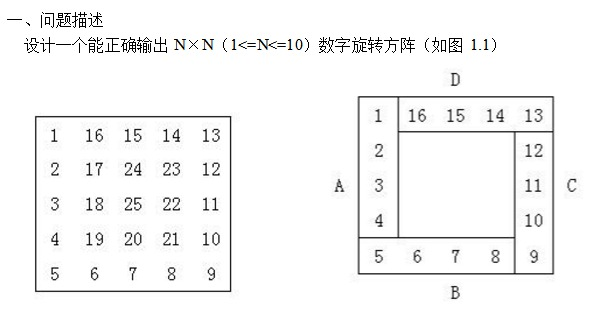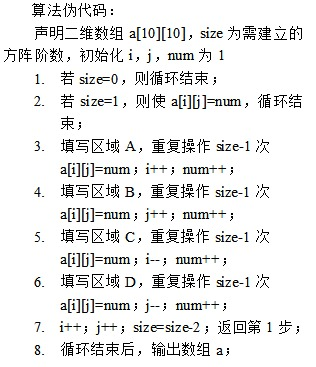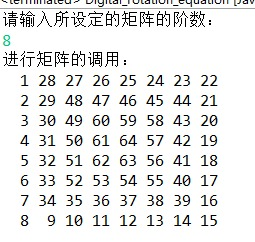## 数字旋转方阵```import java.util.Scanner;

/**
* 数字旋转方程算法
* 每四个区域的一个循环作为一个子问题对象，每次经历一个子问题之后就将问题的规模减2
* 每次子问题开始的第一个元素都按照矩阵的对角线进行选取
*/
public class Digital_rotation_equation {
public static void NMatrix(int n)
{
int size=n;
int flag=1;//用于对子问题当中的每个区域当中所添加数字的个数进行记录
int i=0,j=0,num=1;//num值为向矩阵当中所添加的数字
int[][] array=new int[n][n];
int sum=n*n;//sum用于记录当前矩阵当中还能够添加的元素的个数
int k=n/2+1;//k值代表最大的循环的次数同时也将代表所划分的总共的子问题的个数
int k1=0;//k1+1代表当前正在执行的子问题的个数
while(k1<k)
{
i=k1;j=k1;
if(flag==1)
array[i][j]=num;
//	表示要向当前子问题的A区当中添加的元素的个数为size-1个flag的值表示将要添加的第flag元素值
while(flag<=size-1)
{
/*每次在向矩阵当中添加一个元素的时候sum--,同时要判断当前矩阵当中是否能够继续进行元素的添加，当不能进行添加的
* 时候将会退出循环操作*/
if(sum>0)
{
array[i][j]=num;
sum--;
}
if(sum==0)
break;
//						System.out.println("array["+i+"]["+j+"]="+array[i][j]);
i++;
num++;
flag++;
}
flag=1;
//					开始对B区即最后一行当中的前n-1个位置进行数字的添加
while(flag<=size-1)
{
if(sum>0)
{
array[i][j]=num;
sum--;
}
if(sum==0)
break;
//						System.out.println("array["+i+"]["+j+"]="+array[i][j]);
j++;
flag++;
num++;
}
flag=1;
//					开始对C区进行数字的添加
while(flag<=size-1)
{
if(sum>0)
{
array[i][j]=num;
sum--;
}
if(sum==0)
break;
//						System.out.println("array["+i+"]["+j+"]="+array[i][j]);
num++;
i--;
flag++;
}
flag=1;
//					开始对D区当中进行数字的添加操作
while(flag<=size-1)
{
if(sum>0)
{
array[i][j]=num;
sum--;
}
if(sum==0)
break;
//						System.out.println("array["+i+"]["+j+"]="+array[i][j]);
num++;
j--;
flag++;
}
if(sum==0)
break;
k1++;
size=size-2;
flag=1;
}

//	对最后的矩形进行遍历输出
for (int row= 0; row < array.length; row++) {
for (int low = 0; low < array.length; low++) {
System.out.printf("%3d",array[row][low]);
}
System.out.println("");
}
}
public static void main(String[] args) {
// TODO Auto-generated method stub
System.out.println("请输入所设定的矩阵的阶数：");
Scanner scanner=new Scanner(System.in);
int n=scanner.nextInt();
System.out.println("进行矩阵的调用：");
NMatrix(n);
}
}```